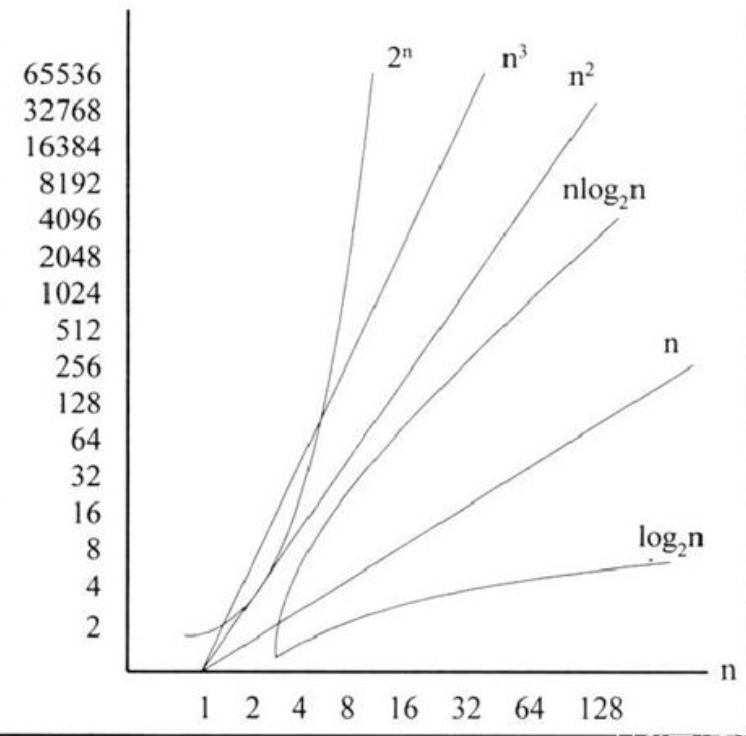+关注继续查看

一、常用算法和复杂度

 算法 名称 复杂度 备注 快速排序 QuickSort(A,p,r) 最坏：O(n2) 平均：O(nlog n) 均衡划分：O(nlog n) 合并排序 MergeSort(A,p,r) O(nlog n) 选最大 FindMax O(n) 选最大和最小 FindMaxMin W(n)=3n/2-2=O(n) 找第二大 锦标赛法 n+logn-2 选择第k小 Select O(n) 动态规划：矩阵连乘 MatrixChain(P,n) 递归：O(2n) 非递归：O(n3) 动态规划：投资问题 O(nm2) m元钱投资n个项目 动态规划：背包问题 目标函数、约束条件、递推方程 动态规划：最长公共子序列 LCS_length(X,Y) LCS(B,i,j) W(mn)=O(mn) S(mn)=O(mn) 动态规划：图像压缩 Compress(P,n) T(n)=O(n) 动态规划：最大子段和 顺序：O(n3) 分支策略：O(nlog n) 动态规划：O(n) 动态规划：凸多边形的三角划分 时间O(n3)，空间O(n2) 动态规划：电路布线 MNS(C,n) T(n)=O(n2)，S(n)=O(n2) 动态规划：最优二叉搜索树 T(n)=O(n2)，S(n)=O(n2) 贪心法：活动选择问题 Greedy Select 贪心法：最优装载问题 Loading O(nlogn) 贪心法：最小延迟调度问题 O(n) 贪心法：找零钱问题 贪心法：装箱问题 贪心法：最优二元前缀码问题 Huffman（C） O(nlogn) 贪心法：文件归并 Huffman算法 O(nlogn) 贪心法：最小生成树 kruskal算法 O(nlogn) 贪心法：单源最短路径 Dijkstra算法 O(n2) 回溯法：四后问题 递归回溯，迭代回溯 四叉树，深度优先 指数级，蒙特卡罗法估计效率 回溯法：最有装载问题二 子集数，深度优先 指数级 分支估界：背包问题 代价函数，深度优先 分支估界：最大团问题 子集树 代价函数：F=Cn+n-k 约束条件 O(n2n) 回溯法：图的m着色问题 O(nmn) 分支估界：巡回售货员问题 O(n!) 分支估界：圆排列问题 O((n+1)!) 分支估界：电路板排列问题 O(mn!) 分支估界：连续邮资问题 指数级

二、动态规划算法

1）  将问题表示成多步判断

2）  确定是否满足优化原则---必要条件

3）  确定子问题的重叠性---估计算法效率

4）  列出关于优化函数的递推方程（或不等式）和边界条件

5）  自底向上计算子问题的优化函数值---非递归的算法

6）  备忘录方法记录中间结果

7）  标记函数追踪问题的解

1）  时间复杂性改进依赖于子问题的重叠程度

2）  空间复杂度较高

三、贪心算法

1）适用于满足优化原则的组合优化问题

2）将问题表示成多步判断

3）确定一个优化测度---贪心选择的依据

4）确定是否满足贪心选择性质---每步贪心选择都会导致最优解

5）自顶向下计算

1）  对步数k归纳

2）  规模k归纳

四、回溯法和分支估界法

搜索问题---约束条件

优化问题---约束条件+代价函数

遍历搜索树的时间，最坏情况为指数，空间代价小

利用对称性裁剪子树

划分成子问题

深度优先、宽度有限、宽深结合、优先函数

五、求解组合优化问题的算法设计技术比较EM算法原理总结
1226 0【算法小总结】二分图的最大独立集
1402 0801 0【算法小总结】母函数模板
1229 01614 0667 02251 05334 0【Java数据结构及算法实战】系列006：算法复杂度等级及其分析

7 01001 0

67

0

《2021云上架构与运维峰会演讲合集》

《零基础CSS入门教程》

《零基础HTML入门教程》